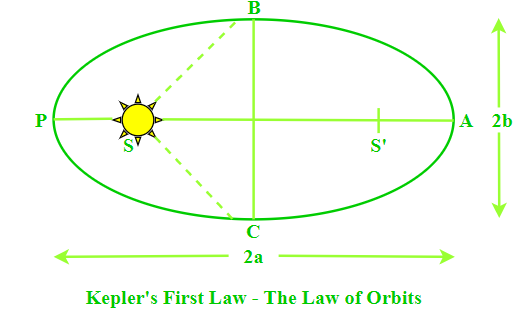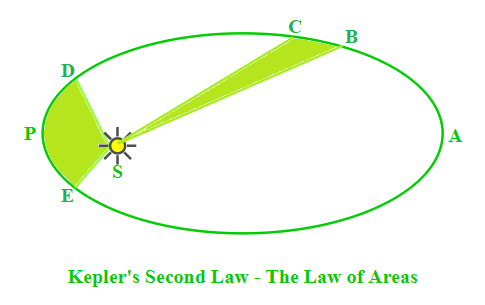Related Articles

# Kepler’s Laws of Planetary Motion

• Last Updated : 30 Jun, 2021

The periods, orbits, and regions of planets and sun are usually the emphasis of Kepler’s Law of planetary motion. In a sun-centered solar system, the planets rotate around the sun in elliptical orbits with the sun at one point. Kepler developed a set of rules based on his assessment of the movements of the planets, which are now known as Kepler’s three laws.

Kepler’s three laws detailed how planets behaved based on their trajectories through space. In 1609, The New Astronomy published the first two of Kepler’s laws of motion. Their finding turned out to be a sincere attempt to advance modern science.

### Introduction to Kepler’s Law

The concept of motion is always relative. Motions are divided into two categories based on the energy of the particle in motion:

Bounded Motion: The particle possesses negative total energy (E < 0) in bounded motion and has two or more extreme points where the total energy is always equal to the particle’s potential energy, i.e. the particle’s kinetic energy becomes zero.

When the eccentricity 0 ≤ e < 1, E < 0 denotes that the body’s motion is bounded. The eccentricity of a circular orbit is zero, whereas the eccentricity of an elliptical orbit is one.

Unbounded Motion: The particle in unbounded motion has positive total energy (E > 0) and a single extreme point where the total energy is always equal to the particle’s potential energy, i.e. the particle’s kinetic energy becomes zero.

When the eccentricity e ≥ 1, E > 0 denotes that the body is moving freely. The eccentricity of a parabolic orbit e = 1, while the eccentricity of a hyperbolic orbit e > 1.

Law of orbits, the law of equal areas, and the law of periods are Kepler’s first, second, and third laws described in detail below.

### Kepler’s First Law — The Law of Orbits

Kepler’s First Law known as the law of ellipses, states that planets revolve around the sun in an elliptical pattern. The law of orbits is a common name for Kepler’s first law.

According to the rule, any planet’s orbit is an ellipse around the Sun, with the Sun at one of the ellipse’s two focal points.

Planets circle the Sun in a circular orbit, which we are already familiar with. Planets do, however, rotate around the Sun, although not in a circular orbit, according to Kepler. However, it is centered on an ellipse.There are two foci (S & S’) in an ellipse. The sun (S) is located at one of the ellipse’s focal points. Perihelion (PS) refers to the point when the planet is closest to the sun, whereas aphelion (AS) refers to the point where the planet is furthest from the sun. The major axis is (2a) and the minor axis is (2b). The total of any planet’s distances from two foci is constant, which is one of the properties of an ellipse. Seasons are brought about by a planet’s elliptical orbit.

### Kepler’s Second Law — The Law of Areas

Kepler’s Second Law, often known as the law of areas, determines the rate at which each planet will spin while circling the sun. The rate at which each planet rotates through space is continuously changing. When a planet is closest to the sun, it spins the quickest, and when it is furthest from the sun, it revolves the slowest.

According to Kepler’s second rule, when the orbiting satellite approaches the focus, it will accelerate. As the orbiting item comes closer to the object pushing it into the orbital path, the gravitational impact on it increases.

The area covered by the planet or spinning object in giving time is the same, regardless of the distance to the item at the focus, according to the mathematical formulation of the law. Because the areas are equivalent, the arc that is further away is shorter, resulting in a slower speed. This holds true for all objects in orbit.The regions developed when the earth is nearest to the sun can be calculated as a broad but short triangle, as shown in the picture below. The regions formed when the earth is furthest from the sun, on the other hand, can be approximated as a narrow but long triangle. These regions are around the same size. When the earth is farthest from the sun, the base of these triangles is the shortest. It has been noticed that for this hypothetical region to have the same size as when the earth is closest to the sun, the earth would have to move more slowly when it is farthest from the Sun.

Law of Conservation of momentum

This law can also be verified by the law of conservation of angular momentum which states that the momentum of a planet revolving around the star in a fixed orbit is always constant along the orbit.

The angular momentum of the planet at position B, LB = m rB vB.

The angular momentum of the planet at position D,  LD = m rD vD.

Now, since L is constant at any point, so LB = LD

⇒ rB vB = rD vD

The above expression shows that the distance and speed are inversely related to each other for a planet revolving in an orbit.

Area enclosed by a planet in an orbit is given by:

dA ⁄ dt = L ⁄ 2 m

where dA is the area enclosed by a planet, dt is the time taken to enclose the area, m is the mass of the planet, and L is the angular momentum of the planet.

### Kepler’s Third Law — The Law of Periods

Kepler’s Third Law, often known as the law of periods, establishes a comparison of a planet’s orbital period and radius of orbit to those of other planets. The 3rd law compares the motion characteristics of various planets, as opposed to Kepler’s 1st and 2nd laws, which describe the motion property of a single planet.

Kepler’s third law defines the relationship between the mass of the planet star system, the distance of planets from the star, and their orbital period. It states that the square of the time period of a planet is directly proportional to the cube of the semi-major axis of the orbit of the planet and inversely proportional to the sum of the mass of the star and planet.

The formula for Kepler’s third law is stated as:

T2 = (4 π2 a3) ⁄ (G (M + m))

where T is the time period, M is the mass of Sun, m is the mass of planet, R is the length of semi-major axis and G is the gravitational constant.

### Sample Problems

Problem 1: Which of the following quantities remain constant in a planetary motion, when seen from the surface of the sun?

When viewed from the inertial frame of the sun, the planets seem to execute elliptical orbital motion with changing kinetic energy (Kepler’s first law) but constant angular momentum due to the force being central.

Problem 2: The radius of a planet is 3 times the radius of the Earth. Calculate the time period of the revolution of the planet.

Solution:

According to Kepler’s third law:

T2 ∝ R3

Consider the radius of the Earth be Re, radius of the planet be Rp, time period of the Earth be Te and time period of the planet be Tp.

Given:

Rp = 3 Re

Time period of the Earth, Te = 1 yr

The relation between the planets is given as:

Tp2 ⁄ Te2 = Rp3 ⁄ Re3

Tp2 ⁄ (1 yr)2 = (3 Re)3 ⁄ Re3

Tp2 = 27 yr2

Tp = 5.2 yr

Hence, the time period of the planet is 5.2 years.

Problem 3: The ratio of orbital angular momentum (about the sun) to the mass of the Earth is 4.4 × 1015 m2 ⁄ s. Find the area enclosed by the Earth.

Solution:

Given:

L ⁄ m = 4.4 × 1015 m2 ⁄ s

Time taken to cover the complete orbit is equal to the time period of the Earth,i.e., dt = 365 days

= 365 × 86400 s

= 31536000 s

Area enclosed by a planet in an orbit is given by:

dA ⁄ dt = L ⁄ 2 m

dA = (L ⁄ m) × (dt ⁄ 2)

= (4.4 × 1015 × 31536000) m2

= 6.938 × 1022 m2

Hence, the area enclosed by the Earth is equal to 6.938 × 1022 m2.

Problem 4: Define Kepler’s First Law.

Kepler’s First Law known as the law of ellipses, states that planets revolves around the sun in an elliptical pattern. The law of orbits is a common name for Kepler’s first law. According to the rule, any planet’s orbit is an ellipse around the Sun, with the Sun at one of the ellipse’s two focal points.

Problem 5: Define the motions of planets based on the energy of the particle in motion.